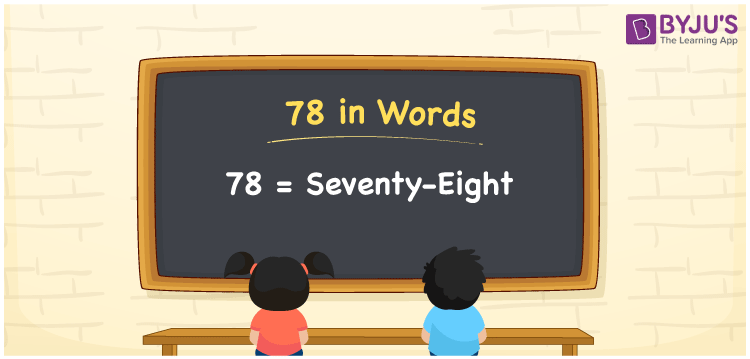# 78 in Words

78 in words is written as Seventy-eight. We can quickly write the two-digit numbers in words since these numbers are very frequently used terms in our daily existence. For example, there are 78 students in class VII, and we say that “Class VII has Seventy-eight students”. In this way, we use the word form of cardinal numbers, i.e., the numbers that denote a certain quantity or amount.

 78 in words Seventy-eight Seventy-eight in Numbers 78

## 78 in English Words

We generally write numbers in words using the English alphabet. Thus, we can read 78 using the words in English as “Seventy-eight”.## How to Write 78 in Words?

Learn how to write 78 in words using the table below that depicts the place value chart for 78.

 Tens Ones 7 8

Here, ones = 8 and tens = 7.

These numbers can be written based on their place values as:

7 × Tens + 8 × Ones

= 7 × 10 + 8 × 1

= 70 + 8

= Seventy + Eight

= Seventy-eight

Therefore, 78 in words = Seventy-eight.

### Facts About the Number 78

78 is a natural number that is the successor of 77 and the predecessor of 79.

78 in words – Seventy-eight

Is 78 an odd number? – No

Is 78 an even number? – Yes

Is 78 a prime number? – No

Is 78 a composite number? – Yes

Is 78 a perfect square number? – No

Is 78 a perfect cube number? – No

How do you write 78 in Roman numerals? – LXXVIII

## Frequently Asked Questions on 78 in Words

Q1

### How do you write 78 in words?

The number 78 can be written in words as seventy-eight.
Q2

### What is the spelling of 78?

The spelling of 78 is Seventy-eight in English words.
Q3

### How to write a cheque for Rs. 78 in words?

A cheque for Rs. 78 in words is written as “Seventy-eight rupees only”.